Question

# An analytical chemist is titrating 241.8mL of a 1.200M solution of nitrous acid HNO2 with a 0.5200M solution of NaOH. Th...

An analytical chemist is titrating 241.8mL of a 1.200M solution of nitrous acid HNO2 with a 0.5200M solution of NaOH. The pKa of nitrous acid is 3.35. Calculate the pH of the acid solution after the chemist has added 605.5mL of the NaOH solution to it.

Note for advanced students: you may assume the final volume equals the initial volume of the solution plus the volume of NaOH solution added.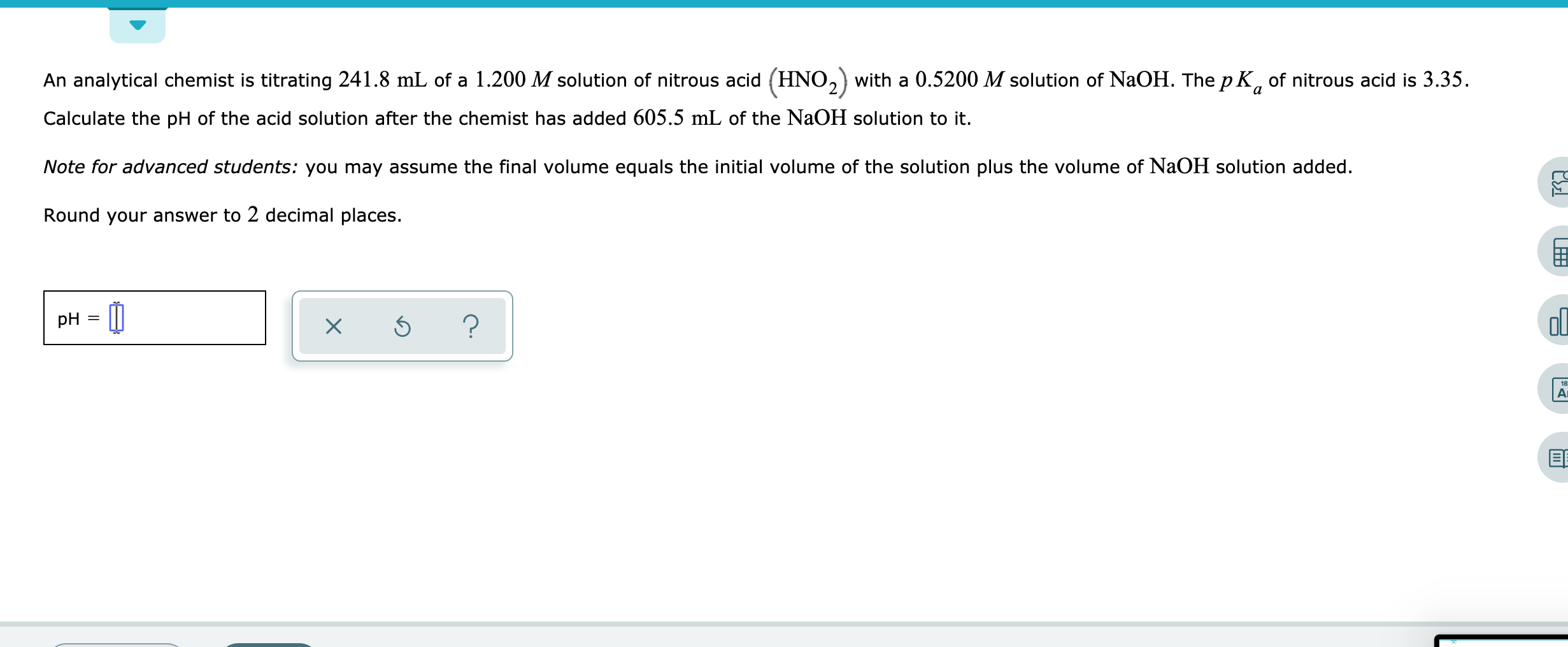An analytical chemist is titrating 241.8 mL of a 1.200 M solution of nitrous acid (HNO2) with a 0.5200 M solution of NaOH. The pK, of nitrous acid is 3.35. Calculate the pH of the acid solution after the chemist has added 605.5 mL of the NaOH solution to it. Note for advanced students: you may assume the final volume equals the initial volume of the solution plus the volume of NaOH solution added. Round your answer to 2 decimal places. p

use:

pKa = -log Ka

3.35 = -log Ka

Ka = 4.467*10^-4

Given:

M(HNO2) = 1.2 M

V(HNO2) = 241.8 mL

M(NaOH) = 0.52 M

V(NaOH) = 605.5 mL

mol(HNO2) = M(HNO2) * V(HNO2)

mol(HNO2) = 1.2 M * 241.8 mL = 290.16 mmol

mol(NaOH) = M(NaOH) * V(NaOH)

mol(NaOH) = 0.52 M * 605.5 mL = 314.86 mmol

We have:

mol(HNO2) = 290.16 mmol

mol(NaOH) = 314.86 mmol

290.16 mmol of both will react

excess NaOH remaining = 24.7 mmol

Volume of Solution = 241.8 + 605.5 = 847.3 mL

[OH-] = 24.7 mmol/847.3 mL = 0.0292 M

use:

pOH = -log [OH-]

= -log (2.915*10^-2)

= 1.5353

use:

PH = 14 - pOH

= 14 - 1.5353

= 12.4647

#### Earn Coins

Coins can be redeemed for fabulous gifts.

Similar Homework Help Questions
• ### An analytical chemist is titrating 91.8 mL of a 0.4200 M solution of nitrous acid (HNO2)...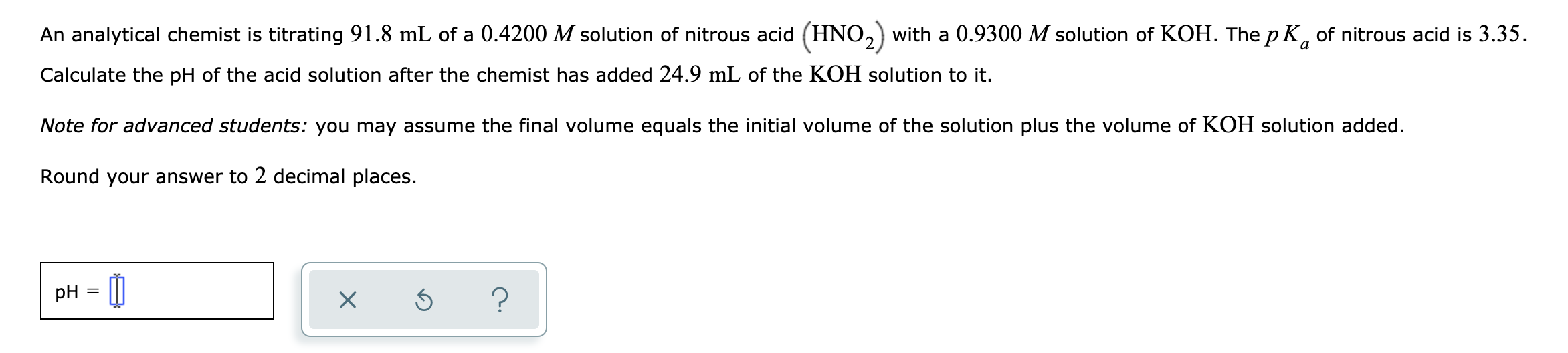An analytical chemist is titrating 91.8 mL of a 0.4200 M solution of nitrous acid (HNO2) with a 0.9300 M solution of KOH. The pK, of nitrous acid is 3.35. Calculate the pH of the acid solution after the chemist has added 24.9 mL of the KOH solution to it. Note for advanced students: you may assume the final volume equals the initial volume of the solution plus the volume of KOH solution added. Round your answer to 2 decimal...

• ### An analytical chemist is titrating 228.6 mL of a 0.3500 M solution of nitrous acid (HNO.)...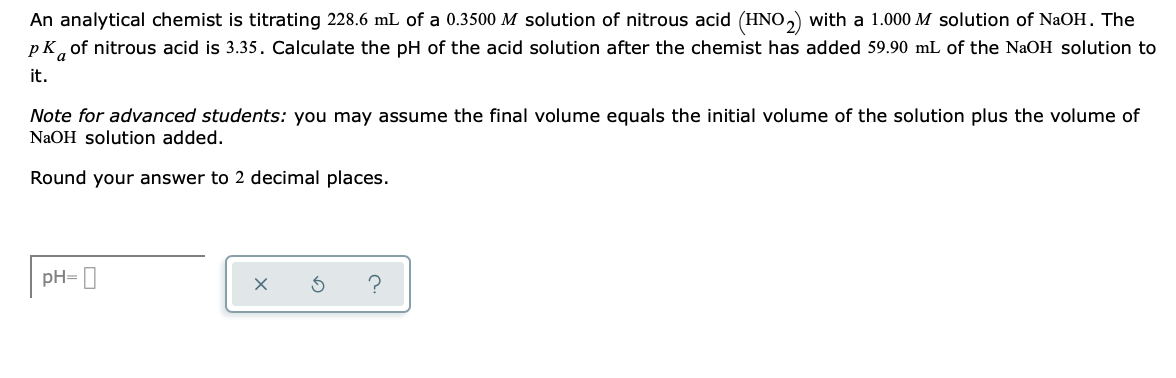An analytical chemist is titrating 228.6 mL of a 0.3500 M solution of nitrous acid (HNO.) with a 1.000 M solution of NaOH. The pk, of nitrous acid is 3.35. Calculate the pH of the acid solution after the chemist has added 59.90 mL of the NaOH solution to it. Note for advanced students: you may assume the final volume equals the initial volume of the solution plus the volume of NaOH solution added. Round your answer to 2 decimal...

• ### An analytical chemist is titrating 118.8 mL of a 0.2500 M solution of nitrous acid (HNO)...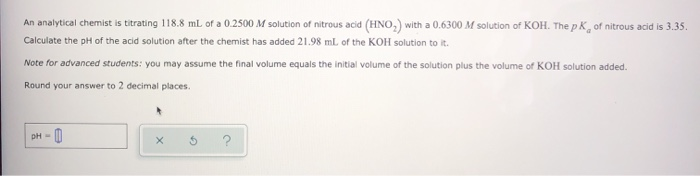An analytical chemist is titrating 118.8 mL of a 0.2500 M solution of nitrous acid (HNO) with a 0.6300 M solution of KOH. The pk of nitrous acid is 3.35. Calculate the pH of the acid solution after the chemist has added 21.98 ml of the KOH solution to it. Note for advanced students: you may assume the final volume equals the initial volume of the solution plus the volume of KOH solution added. Round your answer to 2 decimal...

• ### An analytical chemist is titrating 123.5 mL of a 0.9200 M solution of nitrous acid (HNO...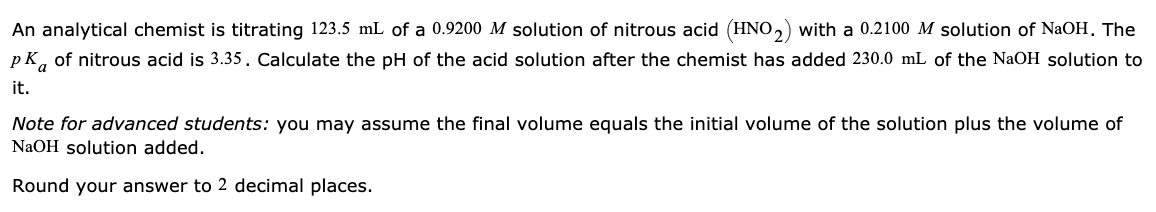An analytical chemist is titrating 123.5 mL of a 0.9200 M solution of nitrous acid (HNO 2) with a 0.2100 M solution of NaOH. The p K, of nitrous acid is 3.35. Calculate the pH of the acid solution after the chemist has added 230.0 mL of the NaOH solution to it. Note for advanced students: you may assume the final volume equals the initial volume of the solution plus the volume of NaOH solution added. Round your answer to...

• ### An analytical chemist is titrating 201.8 mL of a 0.5300 M solution of nitrous acid (HNO, with a 1.100 M solution of KOH...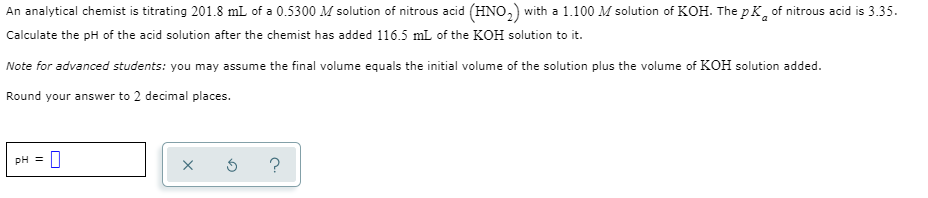An analytical chemist is titrating 201.8 mL of a 0.5300 M solution of nitrous acid (HNO, with a 1.100 M solution of KOH. The pk of nitrous acid is 3.35. Calculate the pH of the acid solution after the chemist has added 116.5 mL of the KOH solution to it. Note for advanced students: you may assume the final volume equals the initial volume of the solution plus the volume of KOH solution added. Round your answer to 2 decimal...

• ### - Calculating the pH of a weak acid titrated with a strong base An analytical chemist is titrating 123.0 ml. of a 0...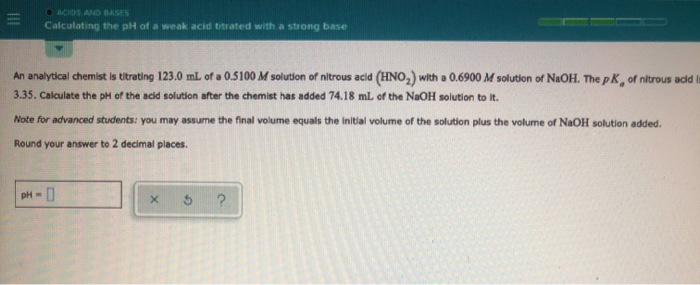- Calculating the pH of a weak acid titrated with a strong base An analytical chemist is titrating 123.0 ml. of a 0.5100 M solution of nitrous acid (HNO,) with a 0.6900 M solution of NaOH. The pk of nitrous add 3.35. Calculate the pH of the acid solution after the chemist has added 74.18 ml of the NaOH solution to it. Note for advanced students you may assume the final volume equals the initial volume of the solution plus...

• ### An analytical chemist is titrating 227.4mL of a 0.5200M solution of ammonia NH3 with a 0.5400M...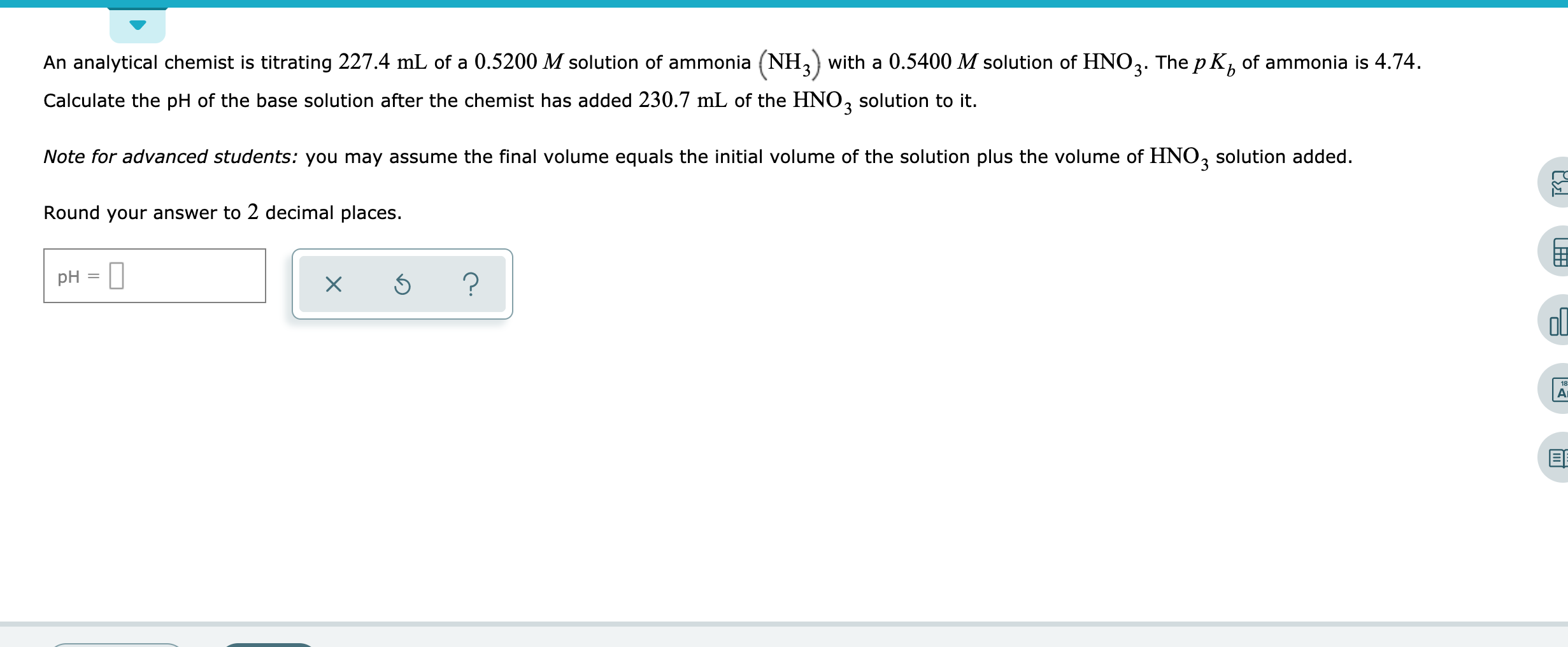An analytical chemist is titrating 227.4mL of a 0.5200M solution of ammonia NH3 with a 0.5400M solution of HNO3. The pKb of ammonia is 4.74. Calculate the pH of the base solution after the chemist has added 230.7mL of the HNO3 solution to it. Note for advanced students: you may assume the final volume equals the initial volume of the solution plus the volume of HNO3solution added. Round your answer to 2 decimal places. An analytical chemist is titrating 227.4...

• ### An analytical chemist is titrating 69.7 mL of a 0.3900 M solution of acetic acid (HCH,...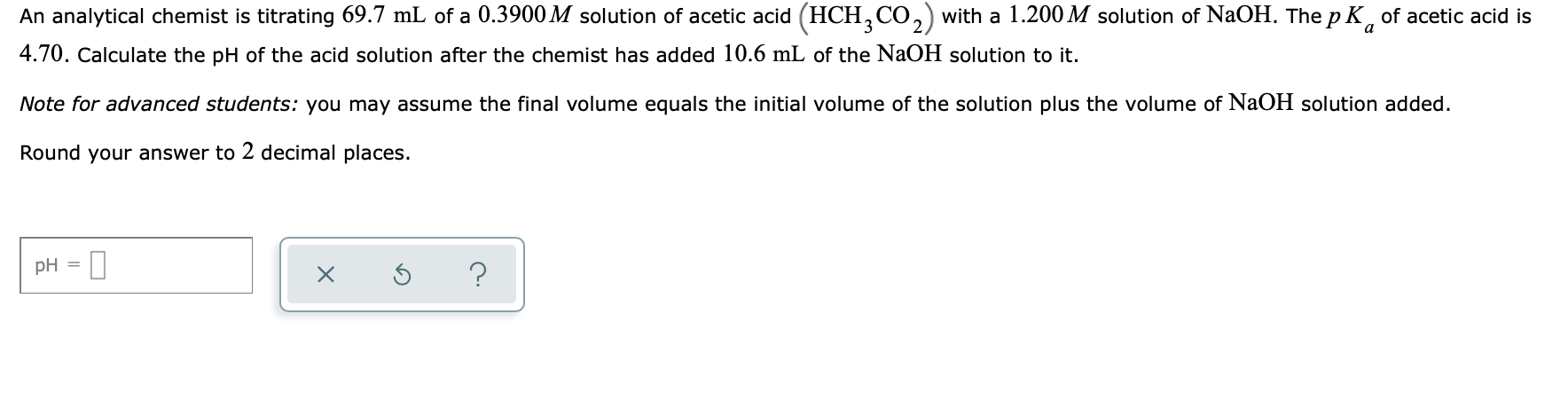An analytical chemist is titrating 69.7 mL of a 0.3900 M solution of acetic acid (HCH, CO,) with a 1.200 M solution of NaOH. The pK, of acetic acid is 4.70. Calculate the pH of the acid solution after the chemist has added 10.6 mL of the NaOH solution to it. Note for advanced students: you may assume the final volume equals the initial volume of the solution plus the volume of NaOH solution added. Round your answer to 2...

• ### An analytical chemist is titrating 195.2mL of a 0.8100M solution of benzoic acid HC6H5CO2 with a...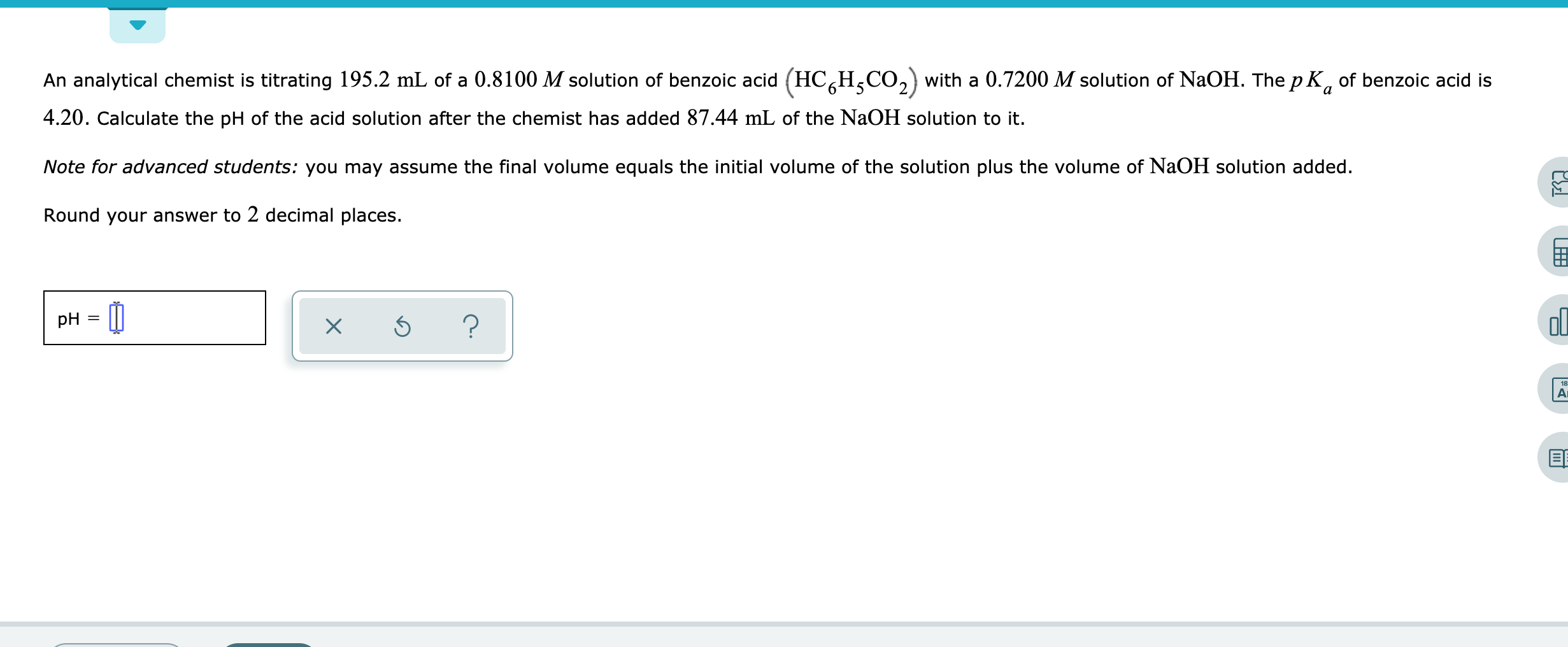An analytical chemist is titrating 195.2mL of a 0.8100M solution of benzoic acid HC6H5CO2 with a 0.7200M solution of NaOH. The pKa of benzoic acid is 4.20. Calculate the pH of the acid solution after the chemist has added 87.44mL of the NaOH solution to it. Note for advanced students: you may assume the final volume equals the initial volume of the solution plus the volume of NaOH solution added. Round your answer to 2 decimal places. An analytical chemist...

• ### An analytical chemist is titrating 238.8 mL of a 1.200 M solution of benzoic acid (HCH...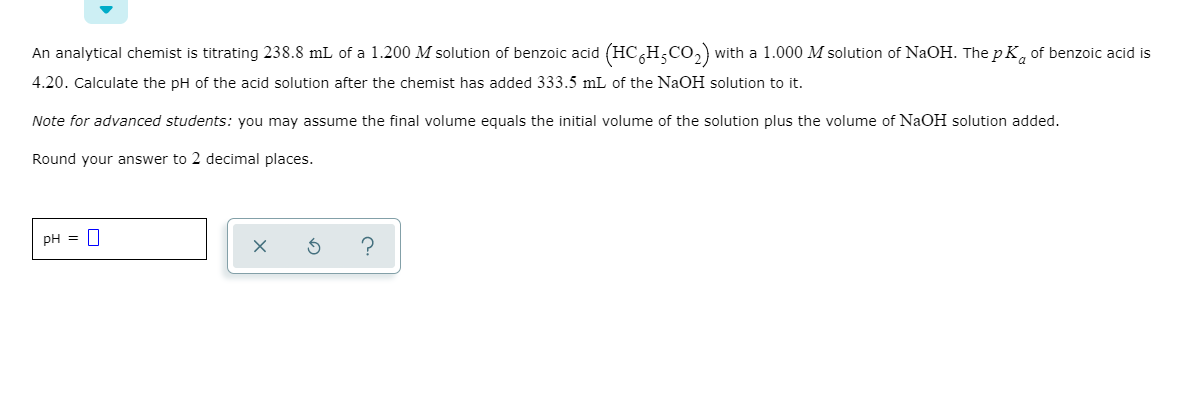An analytical chemist is titrating 238.8 mL of a 1.200 M solution of benzoic acid (HCH CO2) with a 1.000 M solution of NaOH. The pk, of benzoic acid is 4.20. Calculate the pH of the acid solution after the chemist has added 333.5 mL of the NaOH solution to it. Note for advanced students: you may assume the final volume equals the initial volume of the solution plus the volume of NaOH solution added. Round your answer to 2...